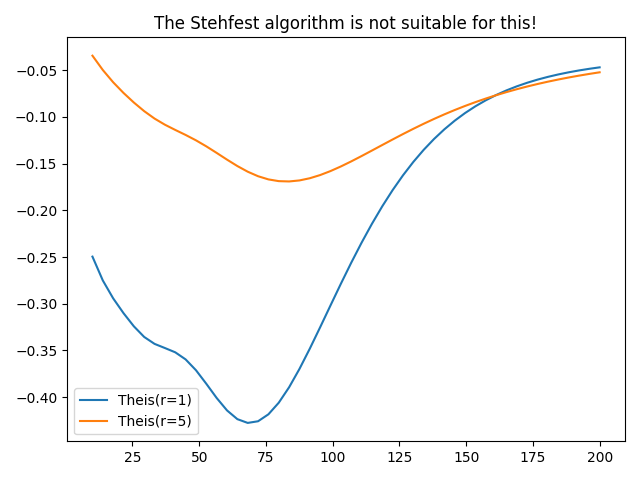# Interval pumping

Another case often discussed in literatur is interval pumping, where the pumping is just done in a certain time frame.

Unfortunatly the Stehfest algorithm is not suitable for this kind of solution, which is demonstrated in the following script.```import numpy as np
from matplotlib import pyplot as plt

from anaflow import theis

time = np.linspace(10, 200)

# Q(t) = Q * characteristic([0, a])
lap_kwargs = {"cond": 3, "cond_kw": {"a": 100}}

time=time,
storage=1e-4,
transmissivity=1e-4,
rate=-1e-4,
lap_kwargs=lap_kwargs,
)